Observing points on the graph

Chapter 3 Class 9 Coordinate Geometry
Concept wise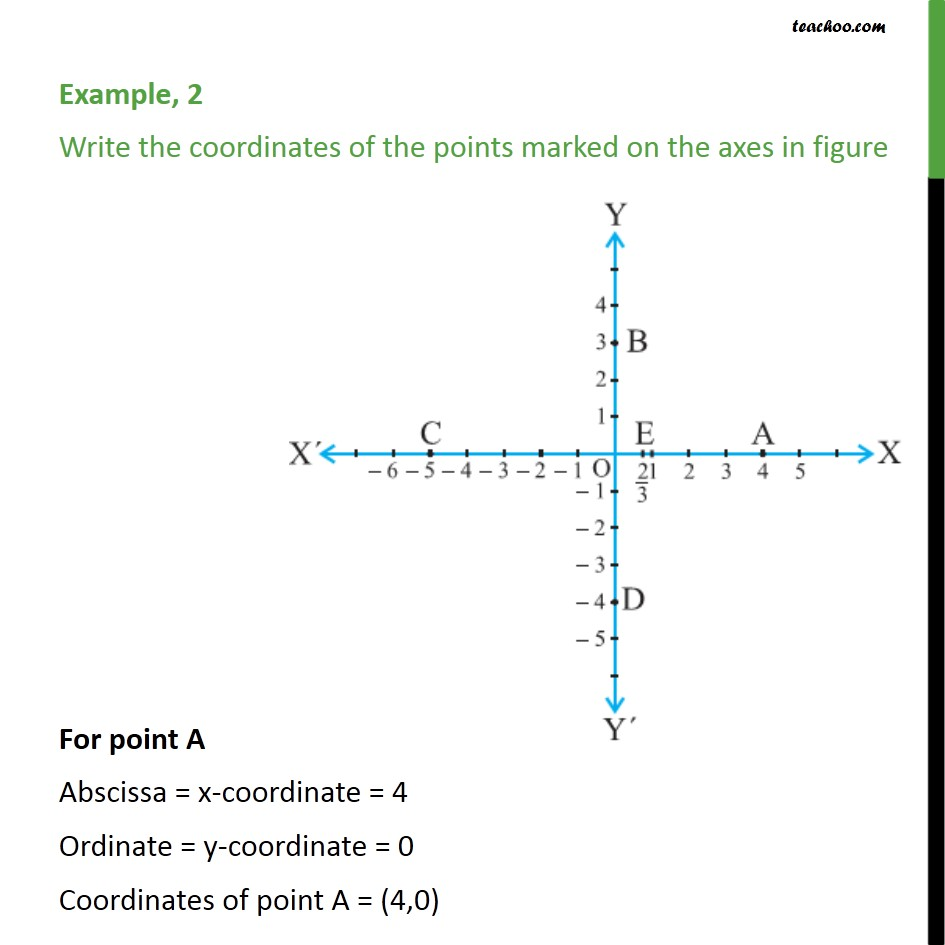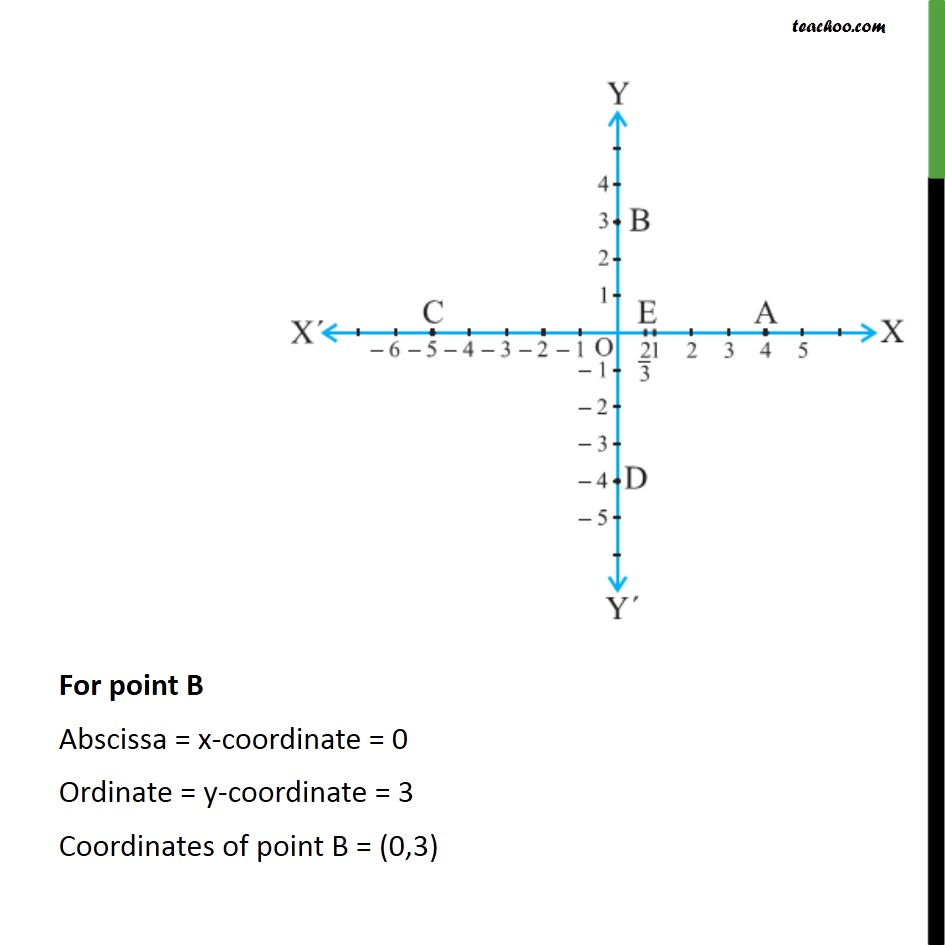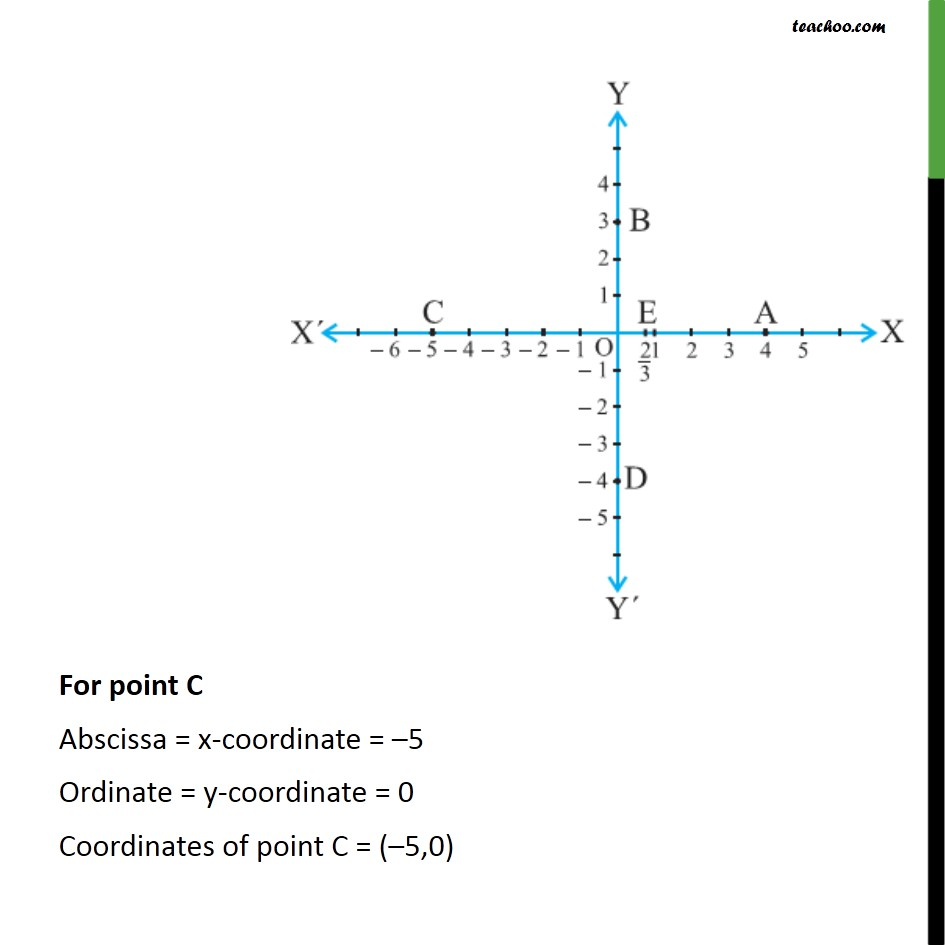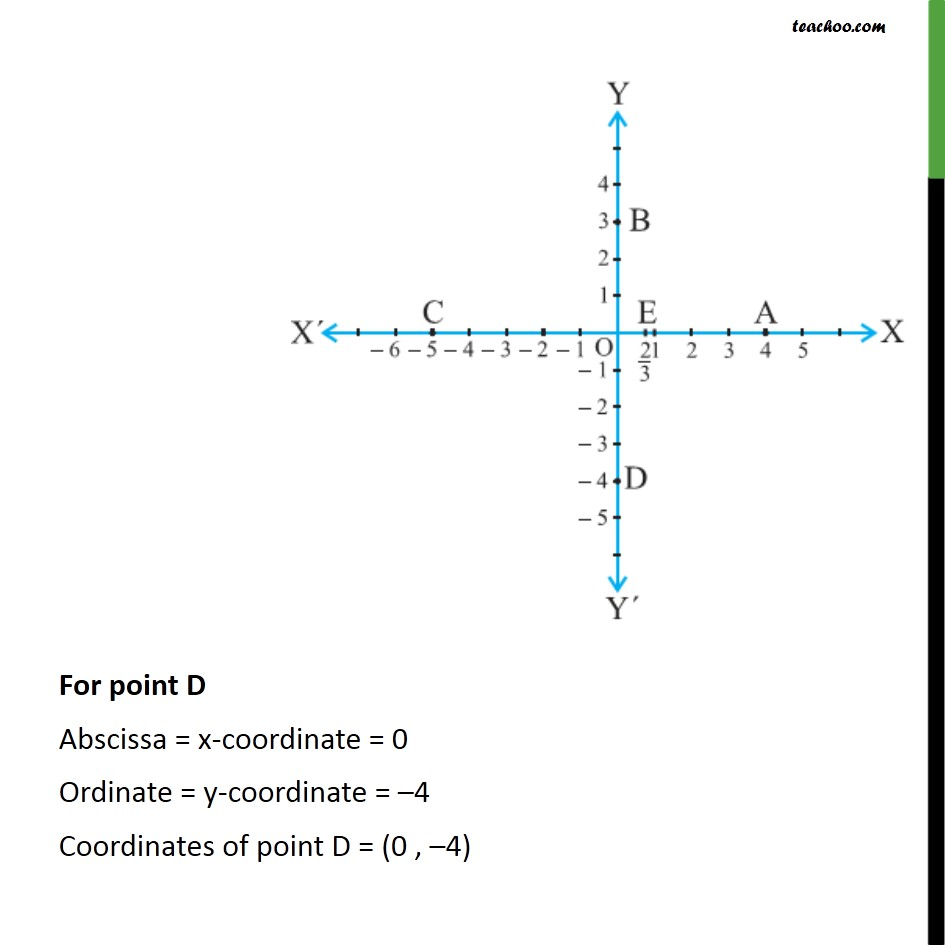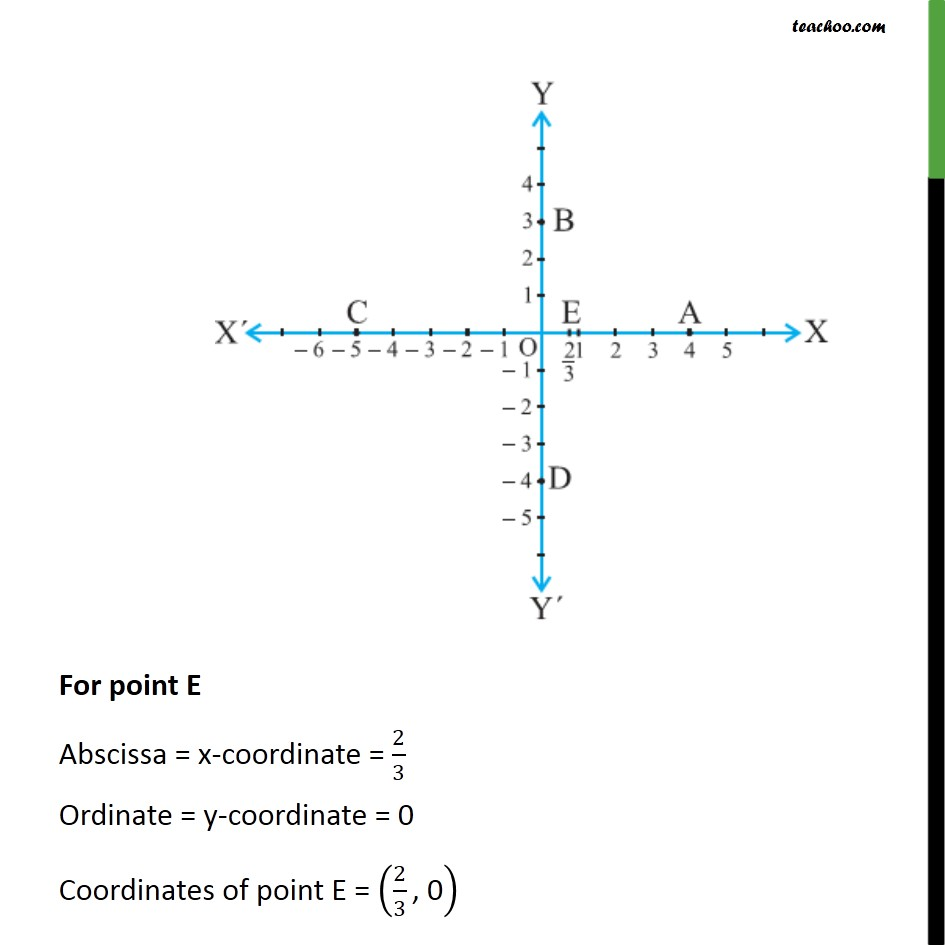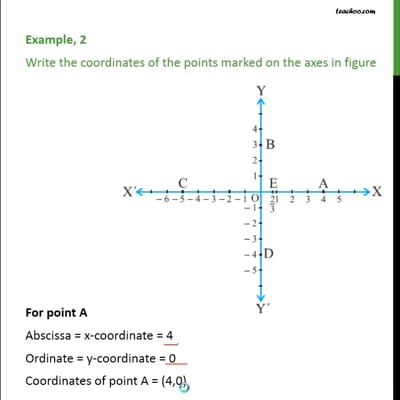This video is only available for Teachoo black users

Introducing your new favourite teacher - Teachoo Black, at only ₹83 per month

### Transcript

Example, 2 Write the coordinates of the points marked on the axes in figure For point A Abscissa = x-coordinate = 4 Ordinate = y-coordinate = 0 Coordinates of point A = (4,0) For point B Abscissa = x-coordinate = 0 Ordinate = y-coordinate = 3 Coordinates of point B = (0,3) For point C Abscissa = x-coordinate = –5 Ordinate = y-coordinate = 0 Coordinates of point C = (–5,0) For point D Abscissa = x-coordinate = 0 Ordinate = y-coordinate = –4 Coordinates of point D = (0 , –4) For point E Abscissa = x-coordinate = 2/3 Ordinate = y-coordinate = 0 Coordinates of point E = (2/3 ", 0" )